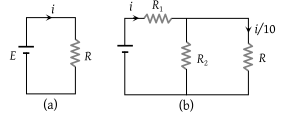# NEET Physics Current Electricity Questions Solved

Consider the circuits shown in the figure. Both the circuits are taking same current from battery but current through R in the second circuit is $\frac{1}{10}$th of current through R in the first circuit. If R is 11 Ω, the value of R1(1) 9.9 Ω

(2) 11 Ω

(3) 8.8 Ω

(4) 7.7 Ω